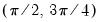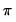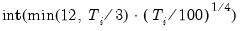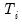seasuroot
Carries out seasonal unit root tests on a series.
Computes Hylleberg, Engle, Granger, and Yoo (HEGY, 1990) test, Smith and Taylor (1998) likelihood HEGY test, Canova and Hansen (CH, 1995), and Taylor (2005) HEGY variance ratio tests.
Syntax
series_name.seasuroot(options)
Options

 type = arg (default= “hegy”) Test type: HEGY (“hegy”), HEGY likelihood (“lklhd”), Canova-Hansen (“ch”), HEGY variance ratio (“hegyvr”). out= arg Output matrix name (optional).
Basic Specification

 periods = arg Periodicity: “2”, “4”, “5”, “6”, or “12”. The default is workfile frequency specific: semi-annual (2), quarterly (4), bi-monthly (6), monthly (12), all others (4). rstrct =arg Restrictions to test (if “test=ch”): arg = space separated list of integers, “all” (all frequencies).For example, if the number of periods is 4, the restriction “0 1 2” corresponds to the 0, harmonic pair, andfrequencies. dtr = arg Non-seasonal deterministic variables to include in estimation: “none” (no exogenous variables), “const” (constant), “trend” (constant and linear trend). The default is “const” for “type=hegyvr” and “none” for all others. sdtr = arg Seasonal deterministic variables to include in estimation: “none” (no seasonal exogenous), “dum” (seasonal dummies), “const” (constant), “trend” (constant and linear trend). lagdep Include lag of dependent variable in computations for Canova-Hansen test (“type = ch”).
Lag Difference Options
Specifies the number of lag difference terms to be included in the test equation. Applicable in “Summary”, LLC, Breitung, IPS, and Fisher-ADF tests. The default setting is to perform automatic lag selection using the Schwarz criteria (“lagmethod=sic”).

 lagmethod=arg (default=“sic”) Method for selecting lag lengths (number of first difference terms) to be included in the Dickey-Fuller test regressions:“aic” (Akaike), “sic” (Schwarz), “hqc” (Hannan-Quinn), “tstat” (Ng-Perron first backward significant t-statistic). lag=arg Specified lag length (number of first difference terms) to be included in the regression: integer (user-specified common lag length), vector_name (user-specific individual lag length, one row per cross-section). maxlag=arg Maximum lag length to consider when performing automatic lag length selection: integer (common maximum lag length), or vector_name (individual maximum lag length, one row per cross-section). The default setting produces individual maximum lags of,default=whereis the length of the cross-section. lagpval=arg (default=0.1) Probability value for use in the t-statistic automatic lag selection method (when “lagmethod = tstat”).
General options

 prompt Force the dialog to appear from within a program. p Print output from the test.
Examples
houst.seasuroot(dtr=trend, sdtr=dum)
runs a traditional HEGY test using seasonal dummies and trend and automatic AIC lag selection.
houst.seasuroot(type=lklhd, dtr=trend, sdtr=trend, info=sic)
runs likelihood HEGY test using spectral intercepts and trends and automatic SIC lag selection.
airline.seasuroot(type=ch, sdtr=const, rstrct=0 6, lagdep)
does Canova-Hansen test for frequency 0 and PI, and add lag of dependent variable as regressor.
Cross-references
See Seasonal Unit Root Testing for a discussion of seasonal unit root testing.